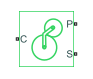# Sun-Planet

Planetary gear set of carrier, planet, and sun wheels with adjustable gear ratio and friction losses

•Libraries:
Simscape / Driveline / Gears / Planetary Subcomponents

## Description

The Sun-Planet gear block represents a set of carrier, planet, and sun gear wheels. The planet is connected to and rotates with respect to the carrier. The planet and sun corotate with a fixed gear ratio that you specify and in the same direction with respect to the carrier. A sun-planet and a ring-planet gear are basic elements of a planetary gear set. For model details, see Equations.

### Thermal Model

You can model the effects of heat flow and temperature change by enabling the optional thermal port. To enable the port, set Friction model to ```Temperature-dependent efficiency```.

### Equations

Ideal Gear Constraints and Gear Ratios

Sun-Planet imposes one kinematic and one geometric constraint on the three connected axes:

`${r}_{\text{C}}{\omega }_{\text{C}}={r}_{\text{S}}{\omega }_{\text{S}}+{r}_{\text{P}}{\omega }_{\text{P}}$`

The planet-sun gear ratio is

`${g}_{\text{PS}}={r}_{\text{P}}/{r}_{\text{S}}={N}_{\text{P}}/{N}_{\text{S}}$`

Where N is the number of teeth on each gear. In terms of this ratio, the key kinematic constraint is:

The three degrees of freedom reduce to two independent degrees of freedom. The gear pair is (1, 2) = (S, P).

Warning

The planet-sun gear ratio gPS must be strictly greater than one.

The torque transfer is:

In the ideal case, there is no torque loss, that is τloss = 0.

Nonideal Gear Constraints and Losses

In the nonideal case, τloss ≠ 0. For more information, see Model Gears with Losses.

### Variables

Use the Variables settings to set the priority and initial target values for the block variables before simulating. For more information, see Set Priority and Initial Target for Block Variables.

## Limitations and Assumptions

• Gear inertia is assumed to be negligible.

• Gears are treated as rigid components.

## Ports

### Conserving

expand all

Rotational conserving port associated with the planet gear carrier.

Rotational conserving port associated with the panet gear.

Rotational conserving port associated with the sun gear.

Thermal conserving port associated with heat flow. Heat flow affects the power transmission efficiency by altering the gear temperatures.

#### Dependencies

To enable this port, set Friction model to `Temperature-dependent efficiency`.

## Parameters

expand all

### Main

Ratio gPS of the planet gear wheel radius to the sun gear wheel radius. This gear ratio must be strictly greater than 1.

### Meshing Losses

Friction model for the block:

• `No meshing losses - Suitable for HIL simulation` — Gear meshing is ideal.

• `Constant efficiency` — Transfer of torque between the gear wheel pairs is reduced by a constant efficiency, η, such that 0 < η ≤ 1.

• `Temperature-dependent efficiency` — Transfer of torque between the gear wheel pairs is defined by the table lookup based on the temperature.

Torque transfer efficiency, ηSP, for sun-planet gear wheel pair meshings. The value must be greater than `0` and less than or equal to `1`.

#### Dependencies

This parameter is exposed when the Friction model parameter is set to ```Constant efficiency```.

Vector of temperatures used to construct a 1-D temperature-efficiency lookup table. The vector elements must increase from left to right.

#### Dependencies

To enable this parameter, set Friction model to `Temperature-dependent efficiency`.

Array of mechanical efficiencies, ratios of output power to input power, for the power flow from the ring gear to the planet gear, ηRP. The block uses the values to construct a 1-D temperature-efficiency lookup table.

Each element is an efficiency that relates to a temperature in the Temperature vector. The length of the vector must be equal to the length of the Temperature vector. Each element in the vector must be in the range (0,1].

#### Dependencies

This parameter is exposed when the Friction model parameter is set to ```Temperature-dependent efficiency```.

Power threshold, pth, above which full efficiency is in effect. Below this values, a hyperbolic tangent function smooths the efficiency factor. For a model without thermal losses, the function lowers the efficiency losses to zero when no power is transmitted. For a model that considers thermal losses, the function smooths the efficiency factors between zero at rest and the values provided by the temperature-efficiency lookup tables at the power thresholds.

#### Dependencies

This parameter is exposed when the Friction model parameter is set to ```Constant efficiency``` or ```Temperature-dependent efficiency```.

### Viscous Losses

Viscous friction coefficient μS for the sun-carrier gear motion.

### Thermal Port

These settings are visible when, in the Meshing Losses settings, the Friction model parameter is set to `Temperature-dependent efficiency`.

Thermal energy required to change the component temperature by a single temperature unit. The greater the thermal mass, the more resistant the component is to temperature change.

#### Dependencies

To enable this parameter, set Friction model to ```Temperature-dependent efficiency```.

expand all

## Version History

Introduced in R2011a